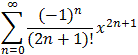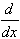#Interactive Real Analysis

Next | Previous | Glossary | Map

## 8.4. Taylor Series

### Proposition 8.4.13: Taylor Series for the Sine Function

sin(x) = x - 1/3! x3 + 1/5! x5 - 1/7! x7 + ... =for all x

The proof is analogous to the proof for the cos-function. Please look up that proof and adjust it to this situation.

### Fun Facts:

1. sin(0) = 0
2. sin is an odd function, i.e. sin(-x) = -sin(x)
3. sin(x -/2) = -cos(x)
4.sin(x) = cos(x)

The fun facts are all easy and are left as exercises. For the third fact you might start with f(x)=cos(x) and develop that in a series around c =/2.

Next | Previous | Glossary | Map